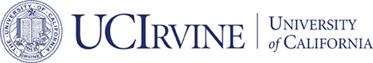# Published## Finite elements for divdiv-conforming symmetric tensors in three dimensions

### Long Chen and Xuehai Huang

#### Mathematics of Computation, 2021

ABSTRACT:

``` Two types of finite element spaces on a tetrahedron are
constructed for divdiv conforming symmetric tensors in three
dimensions. Besides the normal-normal component, another trace
involving combination of first order derivatives of stress should be
continuous across the face. Due to the rigid of polynomials, the
symmetric stress tensor element is continuous at vertices, and on the
plane orthogonal to each edge. Hilbert complex and polynomial
complexes are presented and several decomposition of polynomial vector
and tensors spaces are revealed from the complexes. The constructed
divdiv conforming elements are exploited to discretize the mixed
formulation of the biharmonic equation. Optimal order and
superconvergence error analysis is provided. Hybridization is given
for the ease of implementation.
```